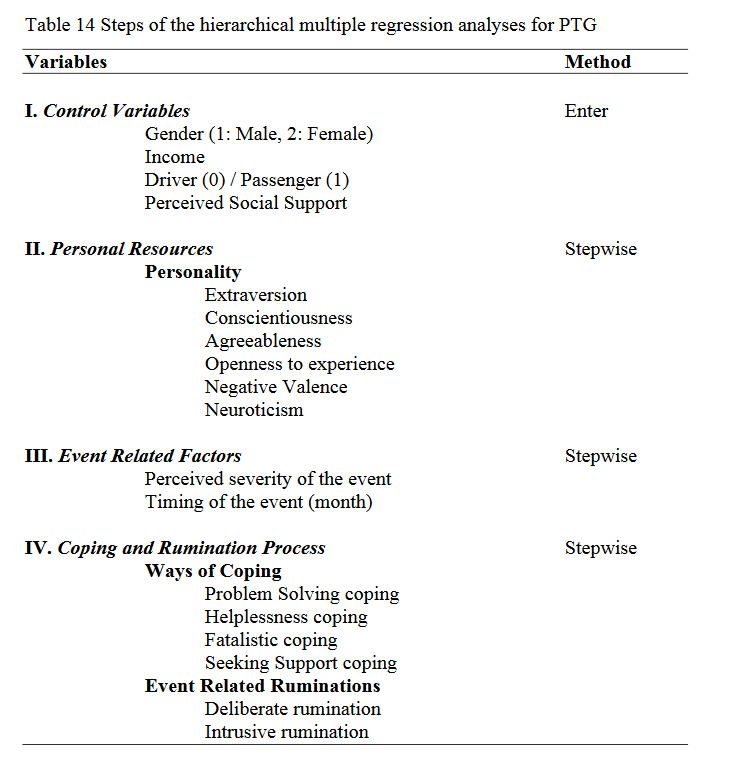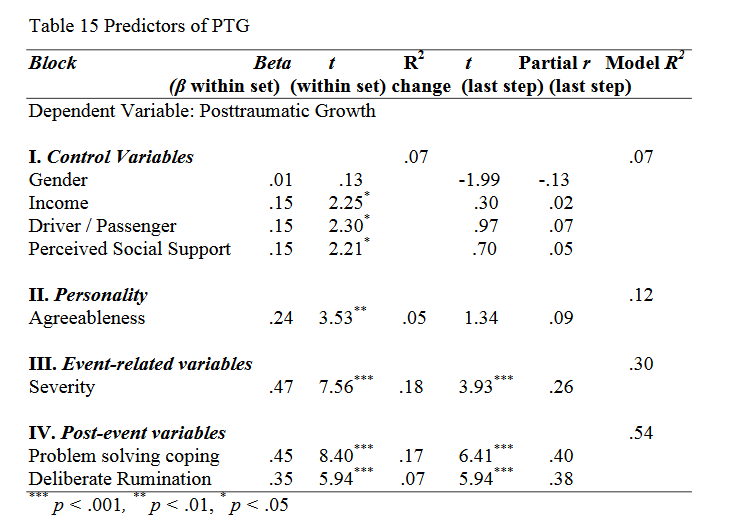Call Choice Point Psychological today: 587-362-0640

A series of hierarchical multiple regression analyses was conducted to examine the predictor variables of posttraumatic growth and to test the Hypothesis 4 (After controlling for the effect of gender, income, being driver or passenger during the accident, and perceived social support; personality variables, perceived severity of the accident, coping, and rumination will predict (i.e explain a significant variance) PTG scores and all of the five domains) of the present study. The variables were entered into the regression equation in four steps. In order to control the effects of gender, income, perceived social support, and being driver or passenger during the accident, these variables were forced to ‘enter’ into the equation in the first step. In the second, third, and fourth steps of the regression, stepwise method was used with the aim of revealing significant associates of PTG. In the second step of the regression, the personality traits (extraversion, conscientiousness, agreeableness, openness to experience, negative valence, and neuroticism) were entered. In the third step, event related factors (timingx and perceived severity of the event) were added to the equation. In the last step, coping and rumination variables (intrusive and deliberate ruminations, helplessness, problem solving, seeking support, and fatalistic ways of coping) were included to the equation (See Table 14).The results of regression analysis revealed that 7% of the variance was explained by control variables. Income (β = .15, t = 2.25, p < .05), being passenger (β = .15, t = 2.30, p < .05), and perceived social support (β = .15, t = 2.21, p < .05) were found to be positively associated with PTG.

In the second step, when personality variables were entered into the equation, only agreeableness positively predicted PTG (β = .24, t = 3.53, p < .01). The contribution of agreeableness enhanced the explained variance to 12% (R2 change = .05, F change (1, 219) = 12.45, p < .01).

In the third step of the regression, perceived severity of the event was positively associated with PTG (β = .47, t = 7.56, p < .001) and by the entrance of this variable, the explained variance reached 30% (R2 change = .18, F change (1, 218) = 57.07, p < .001).

In the last step of the regression equation, with the inclusion of coping and rumination variables, the explained variance of PTG reached 54%. Problem solving coping (β = .45, t = 8.40, p < .001) and deliberate rumination (β = .35, t = 5.94, p < .001) positively predicted PTG.

When all variables of the analysis were in the equation, perceived severity of the event (t = 3.93, p < .001), problem solving coping (t = 6.41, p < .001), and deliberate rumination (t = 5.94, p < .001) were significant predictors, all relating positively to PTG (See Table 15).